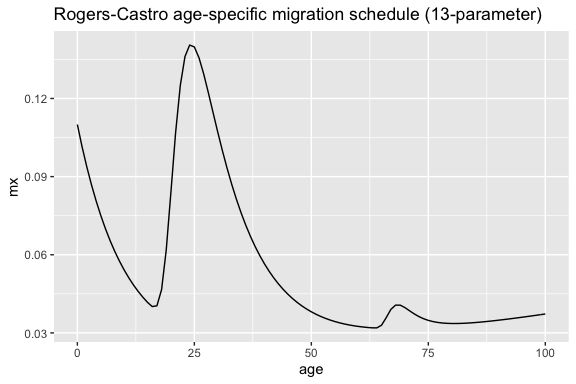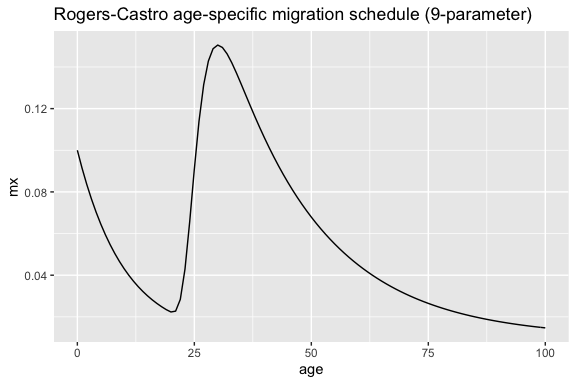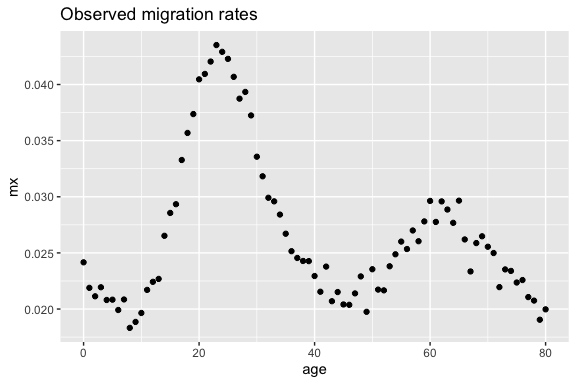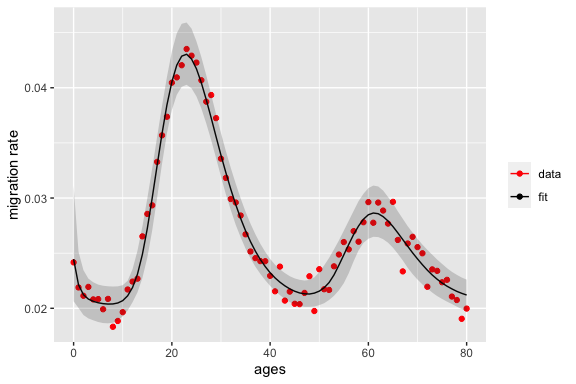# Rogers Castro Migration Models with rcbayes

## Why model migration

Migration happens with multiple transitions over the life course such as entry to education, a new job, or retirement (Preston, Heuveline, and Guillot 2000). These transitions happen more frequently at some ages and come in parallel often with migration. Adult migration usually peaks at young adult ages. Around retirement age, there is a second peak. Due to these regularities, it is possible to model migration by age, which is very important for policymakers and for demographers in estimating population dynamics. Age-specific migration models can help to estimate missing data, smooth noisy data, project trends into the future, and to generalize migration patterns across different populations.

rcbayes has the functionality to fit and estimate age-specific migration schedules based on the Rogers-Castro migration model. This vignette briefly introduces the Rogers-Castro model and then gives examples of both calculating age-specific migration curves given a set of parameters, and fitting the Rogers-Castro model given a set of age-specific migration rates.

## The Rogers and Castro model

Rogers and Castro (1981) developed a mathematical model of migration with up to 13 parameters. Seven of these parameters explain the shape of migration by age, while the rest of parameters represent the intensity of migration. The original formula for the migration rate at age $$x$$ is:

$\begin{equation*} m(x)= a_1 \exp{[ \alpha_1 x ]} + a_2 \exp{[ -\alpha_2 (x - \mu_2) - \exp{ [ -\lambda_2(x - \mu_2) ]} ]}+ a_3 \exp{[ - \alpha_3(x-\mu_3) - \exp{[-\lambda_3 (x-\mu_3)]} ]} + a_4\exp{[\lambda_4x ]}+ c \end{equation*}$

The $$c$$ parameter describes the baseline level of migration. There are four other distinct parts to the equation, which each describe the shape and intensity of migration at different ages:

• pre-working age: $$a_1 \exp{[ \alpha_1 x ]}$$ (Group 1)
• working age: $$a_2 \exp{[ -\alpha_2 (x - \mu_2) - \exp{ [ -\lambda_2(x - \mu_2) ]} ]}$$ (Group 2)
• retirement age: $$a_3 \exp{[ - \alpha_3(x-\mu_3) - \exp{[-\lambda_3 (x-\mu_3)]} ]}$$ (Group 3)
• post-retirement age: $$a_4 \exp{[\lambda_4x ]}$$ (Group 4)

For each of the components, the $$a_k$$ terms describe the heights of the peaks of migration rates. The $$\alpha_k$$ and $$\lambda_k$$ parameters describe the shape of each of the components, in terms of the rate of change over age. And $$\mu_2$$ and $$\mu_3$$ give the ages at the labour force peak and at the retirement peak, respectively.

The migration model need not have all the ‘families’ of migration at different age stages. In practice, there are four combinations of families that are the most common (Rogers, Little, and Raymer 2010):

• The 7 parameter model, which has the pre-working and working age components
• The 9 parameter model, which has the pre-working, working and post-retirement age components
• The 11 parameter model, which has the pre-working, working and retirement age components
• The 13 parameter model, which has all components.

The functions in rcbayes allow for any combination of the components to be included in the model.

## Examples with rcbayes

rcbayes includes two migration model-related functions: mig_calculate_rc, which returns age-specific migration rates calculated based on an age range and set of parameter inputs, and mig_estimate_rc, which estimates parameter values and age-specific migration rates $$m(x)$$ based on an observed age range and migration rates. This section gives examples of both functions.

### Calculating Rogers-Castro migration schedules

We can calculate the implied age-specific rates from a set of parameter inputs. Parameters are defined the same way as in the equation above, that is, c is the overall intensity, the a’s are the intensities at each age family, the alphas and lambdas are the rate of decrease and increase of the shape at each age family, and the mus are the age of peak migration for working age and retirement.

The following is an example specifying values for each of the 13 possible parameters, with values calculated for each age up to age 100:

library(rcbayes)
library(tibble)
library(ggplot2)

pars <- c(a1= 0.09, alpha1= 0.1,
a2= 0.2, alpha2= 0.1, mu2= 21, lambda2= 0.4,
a3= 0.02, alpha3= 0.25, mu3= 67, lambda3= 0.6,
a4= 0.01, lambda4= 0.01,
c= 0.01)

ages <- 0:100
mx <- mig_calculate_rc(ages = ages, pars = pars)

# plot to see what the schedule looks like
df <- tibble(age = ages, mx = mx)
df %>%
ggplot(aes(age, mx)) +
geom_line() +
ggtitle("Rogers-Castro age-specific migration schedule (13-parameter)")Not all parameters need to be specified. The following shows an example of the 9 parameter specification:

pars <- c(a1= 0.09, alpha1= 0.1,
a2= 0.2, alpha2= 0.05, mu2= 25, lambda2= 0.4,
c= 0.01)

ages <- 0:100
mx <- mig_calculate_rc(ages = ages, pars = pars)

# plot to see what the schedule looks like
df <- tibble(age = ages, mx = mx)
df %>%
ggplot(aes(age, mx)) +
geom_line() +
ggtitle("Rogers-Castro age-specific migration schedule (9-parameter)")Note, however, that all parameters within a particular component family must be specified. So for example, if one of the working-age family parameters is specified (Group 2), then all must be specified, otherwise an error occurs.

### Estimating migration age schedules using the Rogers-Castro model

#### Overview

The mig_estimate_rc function returns estimated Rogers-Castro parameters and $$m(x)$$ values, based on observed age-specific migration data and the Rogers-Castro components to be included in the model. The function has the capability of estimating a Rogers-Castro age schedule with any combination of the components pre_working_age, working_age, retirement and post_retirement. These are specified as logicals (either TRUE or FALSE) in the function.

As illustrated above, Rogers-Castro migration age schedules are highly non-linear, as so are not necessarily straight forward to estimate. Previous approaches have used, for example, Excel’s Solver function or the optim function in R.1 However, the estimated parameters and schedules are highly sensitive to the initial values chosen for the parameter values, and convergence is difficult to achieve for the 11 and 13 parameter models.

In rcbayes, we estimate Rogers-Castro schedules in a Bayesian framework using a Markov Chain Monte Carlo (MCMC) algorithm, via the Stan programming language (Carpenter et al. 2017). The use of Bayesian methods allows for priors to be set on parameters, which helps convergence in the estimation process.

##### Required Inputs for mig_estimate_rc

The following arguments are required for the mig_estimate_rc function:

• ages, pre_working_age, working_age, retirement and post_retirement
• Either
• migrants and pop to provide data on the number of age-specific migrants and age-specific population OR
• mx to provide data on age-specific migration rates

That is, users have an option to input their data as counts or as rates. Depending on which one is used, a different model will be run. If the user provides data as counts using migrants and pop, a Poisson model will be applied. If the user provides data as rates, a Normal model will be applied.

When running mig_estimate_rc, a message will appear informing the user of which model is run.

##### Optional Inputs for mig_estimate_rc

In the case that the Normal model is used (i.e., the user inputs age-specific migration rates using the argument mx), the user can use the optional argument sigma to input the standard deviation for the normal model. If this optional input is not used, the value of sigma is estimated.

Regardless of which model is used, any additional arguments for mig_estimate_rc will be additional inputs to rstan::stan().

#### Example: Estimating migration rates for population with large retirement peak

In this example, we will fit an 11-parameter model to a set of observed age-specific rates from a population that resembles 1% of the Florida population in the United States. First, we can plot the observed rates to get a sense of what the age schedule looks like

fl_ages <- 0:80
fl_migrants <- c(49, 48, 48, 52, 50, 45, 42, 46, 45, 44, 47, 55, 57, 59, 67, 69, 71, 78, 93, 88, 116,
106, 102, 104, 102, 123, 112, 102, 112, 105, 100, 83, 81, 77, 78, 77, 66, 64, 65, 64,
68, 52, 59, 51, 54, 55, 52, 58, 64, 53, 68, 53, 57, 67, 71, 78, 75, 77, 77, 83, 88,
80, 84, 79, 77, 83, 71, 59, 65, 67, 64, 63, 56, 50, 43, 46, 46, 38, 32, 28, 29)
fl_pop <- c(2028, 2193, 2271, 2370, 2403, 2160, 2109, 2206, 2456, 2334, 2392, 2534, 2542, 2601, 2526,
2416, 2420, 2344, 2606, 2355, 2867, 2589, 2426, 2390, 2377, 2909, 2753, 2633, 2847, 2819,
2979, 2608, 2708, 2602, 2745, 2883, 2624, 2607, 2677, 2637, 2964, 2414, 2481, 2464, 2510,
2695, 2552, 2711, 2794, 2683, 2888, 2439, 2631, 2814, 2854, 2999, 2959, 2852, 2957, 2985,
2970, 2882, 2839, 2737, 2782, 2799, 2710, 2527, 2512, 2530, 2505, 2521, 2551, 2125, 1838,
2057, 2037, 1804, 1542, 1470, 1452)

df <- tibble(age = fl_ages, mx = fl_migrants / fl_pop)
df %>%
ggplot(aes(age, mx)) +
geom_point() +
ggtitle("Observed migration rates")Let’s fit a Rogers-Castro migration age schedule to these data. Below, we choose to estimate parameters associated with the pre-working age, working and retirement components (but not post retirement). We also provide the data as counts, which means that we are fitting a Poisson model.

rc_res <- mig_estimate_rc(
ages=fl_ages, migrants=fl_migrants, pop=fl_pop,
pre_working_age = TRUE,
working_age = TRUE,
retirement = TRUE,
post_retirement = FALSE,
# (optional) arguments for Stan
chains = 4,
iter = 2000,
control = list(adapt_delta = 0.8, max_treedepth = 10)
)

The mig_estimate_rc function also allows for addition arguments that are related to the Stan model. In the example above, the values listed for chains, iter, adapt_delta and max_treedepth are the default values, so need have not been specified. However, depending on the context, it may make sense to increase the value of each of these components to ensure convergence. More details about these arguments can be found in the R help files for rstan::stan, and also by referring to the Stan documentation.

As mentioned above, this example’s data is in the form of count data. In the case that your data is in the form of migration rates, swap out the migrants and pop arguments for mx.

##### Checking Model Diagnostics

When fitting models in a Bayesian framework using MCMC, as in the case of mig_estimate_rc, one cannot simply run the model and use the results without further inspection. It is always necessary to assess the model results to ensure that the model has converged. In Bayesian models, convergence would imply that the model has converged to a particular target distribution, which is a necessary condition before you move on and use the model’s results.

One measure to check for convergence is to look at the potential scale reduction statistic, commonly referred to as the R-hat statistic (Gelman, Rubin, and others 1992). Ideally, you want to see the R-hat values close to 1 as R-hat values far greater than 1 indicate that convergence has not been achieved. Generally, Gelman et al. recommend ensuring that R-hat values are below 1.1, although there is no universally agreed upon threshold (Gelman et al. 2013). More information about R-hat values is available in the Stan documentation.

In addition to convergence, another difficulty around MCMC algorithms is that the samples may be autocorrelated within a chain. One way to measure this is to look at the effective sample size ($$N_{eff}$$), which tells us the estimation power of your dependent MCMC samples in terms of hypothetical independent samples. A low effective sample size increases the uncertainty of estimates for posterior means, variances, etc (Geyer 2011). If your effective sample size is small, you should consider increasing the number of MCMC samples. More information about the effective sample size is available in the Stan documentation.

The check_converge object in the function output allows you to check the R-hat values and effective sample size.

rc_res[['check_converge']]
#>                    mean      se_mean    n_eff      Rhat
#> alpha1   0.870226739 1.064402e-02 2978.764 0.9994766
#> alpha2   0.190530488 5.443403e-04 1786.985 1.0008539
#> alpha3   0.205526356 1.493772e-03 1706.259 1.0010941
#> a1       0.004726264 6.112932e-05 2180.687 1.0018765
#> a2       0.058373658 1.179189e-04 1821.751 1.0003259
#> a3       0.019992050 1.049587e-04 1671.263 1.0028921
#> mu2     24.985308597 2.063815e-02 2198.856 1.0002768
#> mu3     64.849212229 1.922614e-02 2799.851 1.0007112
#> lambda2  0.140144946 3.200103e-04 1860.819 1.0020920
#> lambda3  0.128113472 6.631156e-04 1639.289 1.0003709
#> c           0.020058990 3.597556e-05 1002.953 1.0015354

In this example, the R-hat values are all close to 1 and effective sample sizes are sufficiently large. We can move on to interpreting the model’s results.

For more details and examples on how to deal with a non-convergent model, please see the rcbayes vignette Achieving Model Convergence With mig_estimate_rc.

##### Examining Model Results

After ensuring that the model has converged properly, you can interpret the results of the model. There are two objects in the function’s output for this purpose.

The pars_df object shows the median estimate and lower and upper bound of a 95% credible interval for the Rogers-Castro parameters. In this example, the working age peak was estimated to be at 24.9 years (95% CI: [23.1, 26.8]).

The fit_df object shows the data and estimated median $$m(x)$$ values at each age $$x$$, along with the lower and upper bound of the 95% credible interval of the fits, and the squared difference between data and the median estimate.

rc_res[['pars_df']]
#> # A tibble: 11 × 4
#>    variable   median     lower   upper
#>    <chr>       <dbl>     <dbl>   <dbl>
#>  1 a1        0.00439  0.000416  0.0111
#>  2 a2        0.0585   0.0485    0.0680
#>  3 a3        0.0200   0.0116    0.0288
#>  4 alpha1    0.752    0.0676    2.26
#>  5 alpha2    0.190    0.148     0.238
#>  6 alpha3    0.196    0.113     0.353
#>  7 c         0.0202   0.0172    0.0219
#>  8 lambda2   0.139    0.116     0.169
#>  9 lambda3   0.125    0.0861    0.191
#> 10 mu2      25.0     23.1      26.9
#> 11 mu3      64.8     62.9      66.8
rc_res[['fit_df']]
#> # A tibble: 81 × 6
#>     ages   data median  lower  upper      diff_sq
#>    <int>  <dbl>  <dbl>  <dbl>  <dbl>        <dbl>
#>  1     0 0.0242 0.0245 0.0206 0.0311 0.0000000885
#>  2     1 0.0219 0.0221 0.0200 0.0251 0.0000000584
#>  3     2 0.0211 0.0212 0.0194 0.0235 0.0000000103
#>  4     3 0.0219 0.0208 0.0191 0.0227 0.00000122
#>  5     4 0.0208 0.0206 0.0189 0.0224 0.0000000306
#>  6     5 0.0208 0.0205 0.0188 0.0222 0.000000109
#>  7     6 0.0199 0.0204 0.0187 0.0220 0.000000257
#>  8     7 0.0209 0.0204 0.0186 0.0220 0.000000219
#>  9     8 0.0183 0.0204 0.0187 0.0220 0.00000430
#> 10     9 0.0189 0.0205 0.0189 0.0220 0.00000266
#> # … with 71 more rows

We can plot the observed data and estimated fit using the fit_df object:

rc_res[["fit_df"]] %>%
ggplot(aes(ages, data)) +
geom_point(aes(color = "data")) +
geom_line(aes(x = ages, y = median, color = "fit")) +
geom_ribbon(aes(ymin = lower, ymax = upper), alpha = 0.2) +
scale_color_manual(name = "", values = c(data = "red", fit = "black")) +
ylab("migration rate")When using mig_estimate_rc it is not unusual to see warnings from Stan, particularly when the retirement and post-retirement families are included in the model. These may include warnings about divergent transitions, low effective sample size and maximum treedepth. If you see these warnings, you should take special care in determining whether your model converged properly.
For more in-depth examples of dealing with warnings and convergence issues, please see the rcbayes vignette Achieving Model Convergence With mig_estimate_rc.